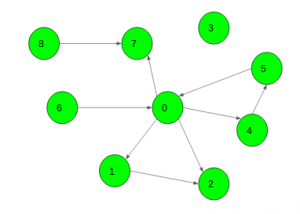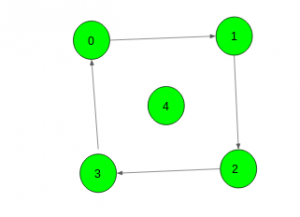# Minimum edges to be added in a directed graph so that any node can be reachable from a given node

Given a directed graph and a node X. The task is to find the minimum number of edges that must be added to the graph such that any node can be reachable from the given node.

Examples:

Input: X = 0Output: 3

Input: X = 4Output: 1

## Recommended: Please try your approach on {IDE} first, before moving on to the solution.

Approach: First, let’s mark all the vertices reachable from X as good, using a simple DFS. Then, for each bad vertex (vertices which are not reachable from X) v, count the number of bad vertices reachable from v (it also can be done by simple DFS). Let this number be cntv. Now, iterate over all bad vertices in non-increasing order of cntv. For the current bad vertex v, if it is still not marked as good, run a DFS from it, marking all the reachable vertices as good, and increase the answer by 1 (in fact, we are implicitly adding the edge (s, v)). It can be proved that this solution gives an optimal answer.

Below is the implementation of the above approach:

## C++

 `// C++ implementation of the approach ` ` `  `#include ` `using` `namespace` `std; ` ` `  `const` `int` `N = 5010; ` ` `  `int` `n, x; ` ` `  `vector<``int``> g[N]; ` ` `  `// To check if the vertex has been ` `// visited or not ` `bool` `vis[N]; ` ` `  `// To store if vertex is reachable ` `// from source or not ` `bool` `good[N]; ` ` `  `int` `cnt; ` ` `  `void` `ADD_EDGE(``int` `u, ``int` `v) ` `{ ` `    ``g[u].push_back(v); ` `} ` ` `  `// Function to find all good vertices ` `void` `dfs1(``int` `v) ` `{ ` `    ``good[v] = ``true``; ` `    ``for` `(``auto` `to : g[v]) ` `        ``if` `(!good[to]) ` `            ``dfs1(to); ` `} ` ` `  `// Function to find cnt of all unreachable vertices ` `void` `dfs2(``int` `v) ` `{ ` `    ``vis[v] = ``true``; ` `    ``++cnt; ` `    ``for` `(``auto` `to : g[v]) ` `        ``if` `(!vis[to] && !good[to]) ` `            ``dfs2(to); ` `} ` ` `  `// Function to return the minimum edges required ` `int` `Minimum_Edges() ` `{ ` ` `  `    ``// Find all vertices reachable from the source ` `    ``dfs1(x); ` ` `  `    ``// To store all vertices with their cnt value ` `    ``vector > val; ` ` `  `    ``for` `(``int` `i = 0; i < n; ++i) { ` ` `  `        ``// If vertex is bad i.e. not reachable ` `        ``if` `(!good[i]) { ` `            ``cnt = 0; ` `            ``memset``(vis, ``false``, ``sizeof``(vis)); ` ` `  `            ``// Find cnt of this vertex ` `            ``dfs2(i); ` `            ``val.push_back(make_pair(cnt, i)); ` `        ``} ` `    ``} ` ` `  `    ``// Sort all unreachable vertices in ` `    ``// non-decreasing order of their cnt values ` `    ``sort(val.begin(), val.end()); ` `    ``reverse(val.begin(), val.end()); ` ` `  `    ``// Find the minimum number of edges ` `    ``// needed to be added ` `    ``int` `ans = 0; ` `    ``for` `(``auto` `it : val) { ` `        ``if` `(!good[it.second]) { ` `            ``++ans; ` `            ``dfs1(it.second); ` `        ``} ` `    ``} ` ` `  `    ``return` `ans; ` `} ` ` `  `// Driver code ` `int` `main() ` `{ ` `    ``// Number of nodes and source node ` `    ``n = 5, x = 4; ` ` `  `    ``// Add edges to the graph ` `    ``ADD_EDGE(0, 1); ` `    ``ADD_EDGE(1, 2); ` `    ``ADD_EDGE(2, 3); ` `    ``ADD_EDGE(3, 0); ` ` `  `    ``cout << Minimum_Edges(); ` ` `  `    ``return` `0; ` `} `

## Java

 `// Java implementation of the approach  ` `import` `java.util.*; ` ` `  `class` `GFG ` `{ ` `     `  `// pair ` `static` `class` `pair ` `{ ` `    ``int` `first,second; ` `    ``pair(``int` `a,``int` `b) ` `    ``{ ` `        ``first = a; ` `        ``second = b; ` `    ``} ` `} ` ` `  `static` `int` `N = ``5010``;  ` ` `  `static` `int` `n, x;  ` ` `  `static` `Vector> g = ``new` `Vector>();  ` ` `  `// To check if the vertex has been  ` `// visited or not  ` `static` `boolean` `vis[] = ``new` `boolean``[N];  ` ` `  `// To store if vertex is reachable  ` `// from source or not  ` `static` `boolean` `good[] = ``new` `boolean``[N];  ` ` `  `static` `int` `cnt;  ` ` `  `static` `void` `ADD_EDGE(``int` `u, ``int` `v)  ` `{  ` `    ``g.get(u).add(v);  ` `}  ` ` `  `// Function to find all good vertices  ` `static` `void` `dfs1(``int` `v)  ` `{  ` `    ``good[v] = ``true``;  ` `    ``for` `(``int` `to = ``0``; to < g.get(v).size(); to++)  ` `        ``if` `(!good[g.get(v).get(to)])  ` `            ``dfs1(g.get(v).get(to));  ` `}  ` ` `  `// Function to find cnt of all unreachable vertices  ` `static` `void` `dfs2(``int` `v)  ` `{  ` `    ``vis[v] = ``true``;  ` `    ``++cnt;  ` `    ``for` `(``int` `to = ``0``; to < g.get(v).size(); to++)  ` `        ``if` `(!vis[g.get(v).get(to)] && !good[g.get(v).get(to)])  ` `            ``dfs2(g.get(v).get(to));  ` `}  ` ` `  `// Function to return the minimum edges required  ` `static` `int` `Minimum_Edges()  ` `{  ` ` `  `    ``// Find all vertices reachable from the source  ` `    ``dfs1(x);  ` ` `  `    ``// To store all vertices with their cnt value  ` `    ``Vector val = ``new` `Vector();  ` ` `  `    ``for` `(``int` `i = ``0``; i < n; ++i)  ` `    ``{  ` ` `  `        ``// If vertex is bad i.e. not reachable  ` `        ``if` `(!good[i])  ` `        ``{  ` `            ``cnt = ``0``;  ` `            ``for``(``int` `j = ``0``; j < vis.length; j++) ` `                ``vis[j] = ``false``; ` ` `  `            ``// Find cnt of this vertex  ` `            ``dfs2(i);  ` `            ``val.add(``new` `pair(cnt, i));  ` `        ``}  ` `    ``}  ` ` `  `    ``// Sort all unreachable vertices in  ` `    ``// non-decreasing order of their cnt values  ` `    ``Collections.sort(val,``new` `Comparator() ` `    ``{  ` `            ``public` `int` `compare(pair p1, pair p2)  ` `            ``{  ` `                ``return` `p1.first - p2.first;  ` `            ``}  ` `    ``});  ` `         `  `    ``Collections.reverse(val);  ` ` `  `    ``// Find the minimum number of edges  ` `    ``// needed to be added  ` `    ``int` `ans = ``0``;  ` `    ``for` `(``int` `it = ``0``; it < val.size(); it++)  ` `    ``{  ` `        ``if` `(!good[val.get(it).second])  ` `        ``{  ` `            ``++ans;  ` `            ``dfs1(val.get(it).second);  ` `        ``}  ` `    ``}  ` ` `  `    ``return` `ans;  ` `}  ` ` `  `// Driver code  ` `public` `static` `void` `main(String args[]) ` `{  ` `    ``// Number of nodes and source node  ` `    ``n = ``5``; x = ``4``;  ` `     `  `    ``for``(``int` `i = ``0``; i < N + ``1``; i++) ` `    ``g.add(``new` `Vector()); ` ` `  `    ``// Add edges to the graph  ` `    ``ADD_EDGE(``0``, ``1``);  ` `    ``ADD_EDGE(``1``, ``2``);  ` `    ``ADD_EDGE(``2``, ``3``);  ` `    ``ADD_EDGE(``3``, ``0``);  ` ` `  `    ``System.out.println( Minimum_Edges());  ` `} ` `}  ` ` `  `// This code is contributed by Arnab Kundu `

## Python3

 `# Python3 implementation of the approach ` `N ``=` `5010` `g ``=` `[[] ``for` `i ``in` `range``(N)] ` ` `  `# To check if the vertex  ` `# has been visited or not ` `vis ``=` `[``False` `for` `i ``in` `range``(N)] ` ` `  `# To store if vertex is reachable ` `# from source or not ` `good ``=` `[``False` `for` `i ``in` `range``(N)] ` ` `  `def` `ADD_EDGE(u, v): ` ` `  `    ``g[u].append(v) ` ` `  `# Function to find all good vertices ` `def` `dfs1(v): ` ` `  `    ``good[v] ``=` `True` `    ``for` `to ``in` `g[v]: ` `        ``if` `not` `good[to]: ` `            ``dfs1(to) ` ` `  `# Function to find cnt of ` `# all unreachable vertices ` `def` `dfs2(v): ` `     `  `    ``global` `cnt ` `    ``vis[v] ``=` `True` `    ``cnt ``+``=` `1` `    ``for` `to ``in` `g[v]: ` `        ``if` `not` `vis[to] ``and` `not` `good[to]: ` `            ``dfs2(to) ` ` `  `# Function to return   ` `# the minimum edges required ` `def` `Minimum_Edges(): ` ` `  `    ``global` `vis, cnt ` `     `  `    ``# Find all vertices reachable ` `    ``# from the source ` `    ``dfs1(x) ` ` `  `    ``# To store all vertices  ` `    ``# with their cnt value ` `    ``val ``=` `[] ` ` `  `    ``for` `i ``in` `range``(``0``, n):  ` ` `  `        ``# If vertex is bad i.e. not reachable ` `        ``if` `not` `good[i]: ` `            ``cnt ``=` `0` `            ``vis ``=` `[``False` `for` `i ``in` `range``(N)] ` ` `  `            ``# Find cnt of this vertex ` `            ``dfs2(i) ` `            ``val.append((cnt, i)) ` ` `  `    ``# Sort all unreachable vertices  ` `    ``# in non-decreasing order of ` `    ``# their cnt values ` `    ``val.sort(reverse ``=` `True``) ` ` `  `    ``# Find the minimum number of edges ` `    ``# needed to be added ` `    ``ans ``=` `0` `    ``for` `it ``in` `val:  ` `        ``if` `not` `good[it[``1``]]:  ` `            ``ans ``+``=` `1` `            ``dfs1(it[``1``]) ` ` `  `    ``return` `ans ` ` `  `# Driver code ` `if` `__name__ ``=``=` `"__main__"``: ` ` `  `    ``# Number of nodes and source node ` `    ``n, x ``=` `5``, ``4` ` `  `    ``# Add edges to the graph ` `    ``ADD_EDGE(``0``, ``1``) ` `    ``ADD_EDGE(``1``, ``2``) ` `    ``ADD_EDGE(``2``, ``3``) ` `    ``ADD_EDGE(``3``, ``0``) ` ` `  `    ``print``(Minimum_Edges()) ` ` `  `# This code is contributed by Rituraj Jain `

Output:

```1
```My Personal Notes arrow_drop_uppawanasipugmailcom

If you like GeeksforGeeks and would like to contribute, you can also write an article using contribute.geeksforgeeks.org or mail your article to contribute@geeksforgeeks.org. See your article appearing on the GeeksforGeeks main page and help other Geeks.

Please Improve this article if you find anything incorrect by clicking on the "Improve Article" button below.

Improved By : rituraj_jain, andrew1234